Latest Results:

11 Plus Area and Perimeter

The area is the place occupied by any object. Area and perimeter are measurements of an object. The area always expressed in square units. Perimeter is expressed in units. Here we will learn about the area and perimeter of the following two-dimensional shapes:

1. Square

2. Rectangle

3. Triangle

4. Circle

5. Parallelogram

6. Trapezium

7. Rhombus

Area and perimeter of a Square:

The place occupied by a square is called the area of a square.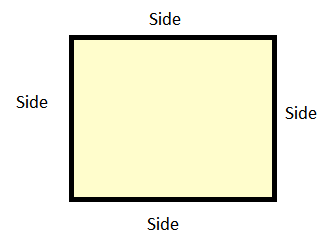The area of square = side x side (square units).

Example: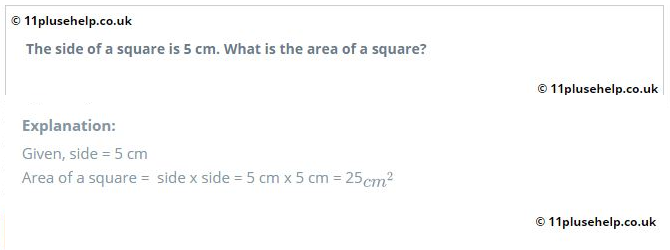The perimeter of square = 4 x side (unit)

Area  and perimeter of rectangle:

The place occupied by a rectangle is called the area of the rectangle.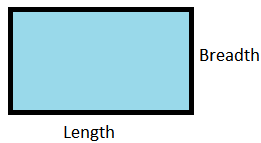The area of rectangle = length x breadth  (square units).

The perimeter of Rectangle = 2 x (length + breadth) (unit)

Example: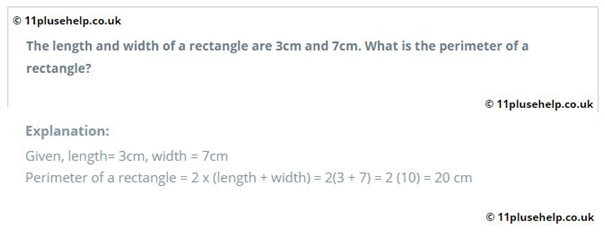Area  and perimeter of a triangle:

The place occupied by a triangle is called the area of the triangle.The area of triangle = 12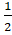x base x Height (square units).

Example: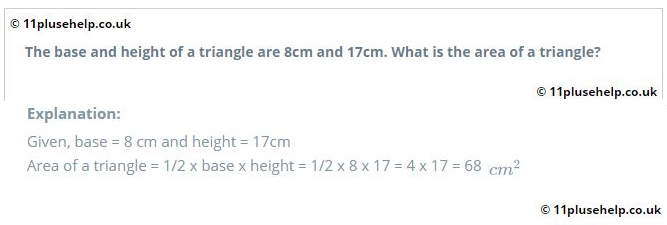The area of the equilateral triangle = 34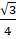a2 (square units).

a = side of a triangle

The perimeter of Triangle = (AB + BC + CA) (unit)

Area and circumference of a circle: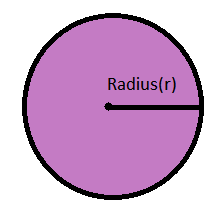r = radius of a circle

Diameter(d) = 2r

r = d/2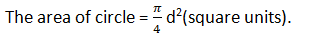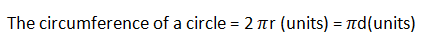Example: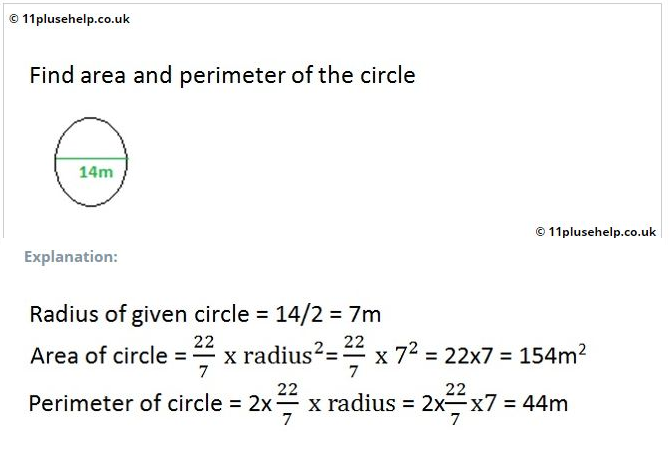Area and perimeter of parallelogram:

The place occupied by a parallelogram is called the area of a parallelogram.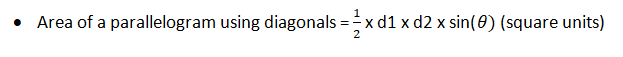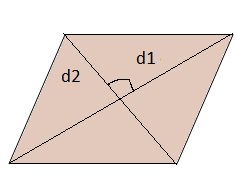d1 and d2 are the lengths of two diagonals of a parallelogram.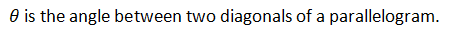• Area of a parallelogram using base and height = base x height(square units)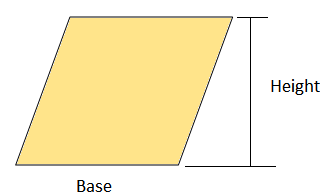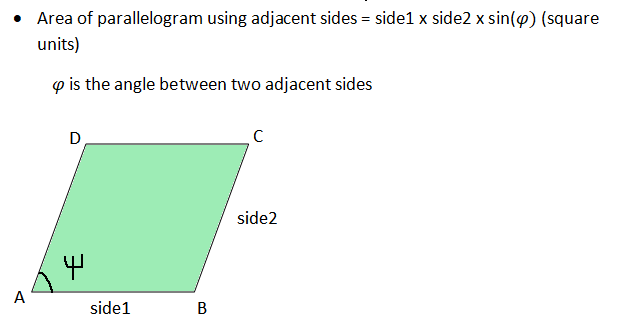The perimeter of parallelogram =2 (side1 + side2) (units)

Example: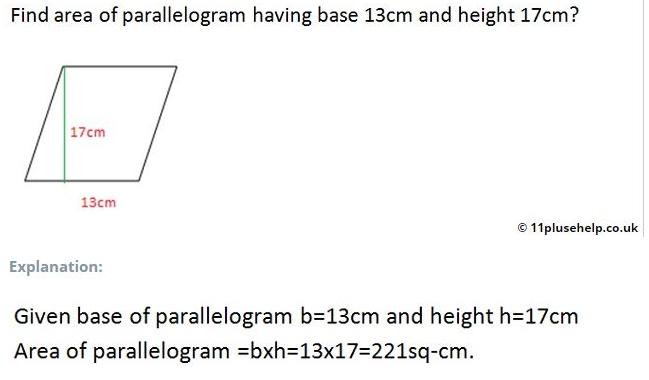Area and perimeter of trapezium:

The place occupied by the trapezium is called the area of trapezium.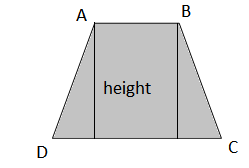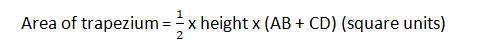The perimeter of trapezium = (AB + BC + CD + DA) (units)

Area and perimeter of rhombus:

The place occupied by the rhombus is called the area of the rhombus.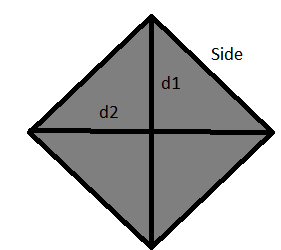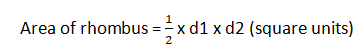d1 and d2 are the lengths of two diagonals of a rhombus.

Example: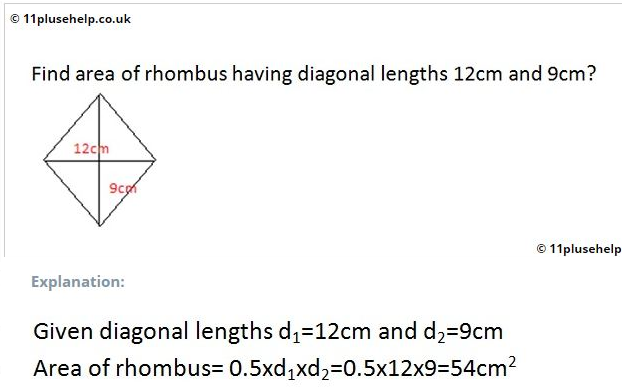The perimeter of rhombus =  4(side) (units)

Example: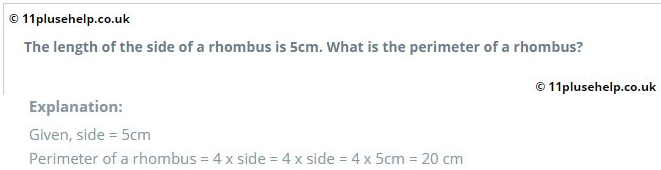11plusehelp.co.uk  provides different types of problems on each 11 plus maths topics with detailed explanations. It provides maths past papers in the form of multiple-choice or blank type practice paper with time-bound. We provide word problems on maths topics like addition subtraction, skip counting, age range, pre-algebra, fraction decimals, etc.11plusehelp.co.uk is useful to increase students' math skills. We provided flashcards to revise the subjects.

Our platform 11PluseHelp.co.uk is a one-stop shop for 11 plus preparation for children. We also help parents and tutors to make your child prepare for the test.

At 11plusehelp.co.uk/, we possess the following resources:

Maths- 11 Plus Maths Worksheets, 11 Plus Maths Papers free download, 11 Plus Maths topics pdfs.

English- 11 Plus English papers with answers, 11 Plus comprehension with answers, 11 English comprehensions multiple-choice, English reasoning test.

Verbal and Non-Verbal Reasoning- 11 Plus Verbal Reasoning worksheets free, Verbal and Non-Verbal Reasoning tests, easy Non-Verbal Reasoning Worksheets

Practice Tests- Eleven plus sample papers, free 11 plus papers with answers, 11 plus mock test papers pdf, 11 exam question and answers, UK 11 plus exam questions, 11 online tests in Maths and English with 11 Plus answers.

To access Virtual Mock Exams, please visit:

https://11plus-mockexams.co.uk/

You can access 11 Plus FREE Papers by visiting the below link:
https://www.11plusehelp.co.uk/11-plus-free-online-papers

You can access 11 Plus FREE Sample Papers by visiting the below link:
https://www.11plusehelp.co.uk/11-plus-sample-papers

11 Plus Complete solution featureshttps://11plusehelp.co.uk/blog/2017/05/01/11-plus-complete-solution/

Practice and Perseverance Over Genius and Talent

Thanks,

11Plusehelp.co.uk